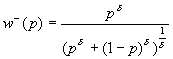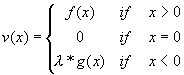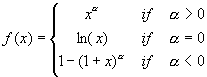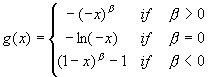Program for Calculating the Cumulative-Prospect-Theory Value
of Prospects with at Most Four Outcomes

by Veronika Köbberling, reproduced here with permission of the author

The program calculates the value of prospects with no more than four outcomes under cumulative prospect theory (CPT), the theory introduced by

Tversky, Amos & Daniel Kahneman (1992), "Advances in Prospect Theory: Cumulative Representation of Uncertainty," Journal of Risk and Uncertainty, 5, 297-323.

You can replace the outcomes, probabilities, and parameters by the ones that you want to consider. The CPT value and the certainty equivalent of the prospect under consideration will be given, as well as the decision weights of the outcomes.

Parameters:
 a: (power for gains; 0.88 in T&K). b: (power for losses; 0.88 in T&K). l: (loss aversion; 2.25 in T&K). g: (probability weighting parameter for gains; 0.61 in T&K). d: (probability weighting parameter for losses; 0.69 in T&K).

 First Outcome: with Probability: Decision Weight of Outcome 1:Second Outcome: with Probability: Decicion Weight of Outcome 2: Third Outcome: with Probability: Decision Weight of Outcome 3: Fourth Outcome: with Probability: Decision Weight of Outcome 4: CPT-Value: Certainty Equivalent:

Elucidation of the Parametric Families:
 The weighting function for gains is given below: and The weighting function for losses is shown below:The value function (where loss aversion parameter is l) is as follows:where f(x) and g(x) are defined as follows:andwritten by Veronika Köbberling, November 2002